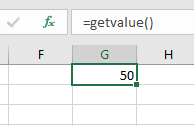# VBA Function – Call, Return Value, & Parameters

This tutorial will teach you to create and use functions with and without parameters in VBA

VBA contains a large amount of built-in functions for you to use, but you are also able to write your own.   When you write code in VBA, you can write it in a Sub Procedure, or a Function Procedure. A Function Procedure is able to return a value to your code.  This is extremely useful if you want VBA to perform a task to return a result. VBA functions can also be called from inside Excel, just like Excel’s built-in Excel functions.

## Creating a Function without Arguments

To create a function you need to define the function by giving the function a name. The function can then be defined as a data type indicating the type of data you want the function to return.

You may want to create a function that returns a static value each time it is called – a bit like a constant.

``````Function GetValue() As Integer
GetValue = 50
End Function``````

If you were to run the function, the function would always return the value of 50.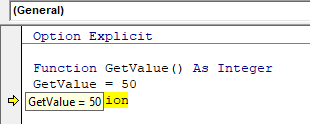You can also create functions that refer to objects in VBA but you need to use the Set Keyword to return the value from the function.

``````Function GetRange() as Range
Set GetRange = Range("A1:G4")
End Function``````

If you were to use the above function in your VBA code, the function would always return the range of cells A1 to G4 in whichever sheet you are working in.

## Calling a Function from a Sub Procedure

Once you create a function, you can call it from anywhere else in your code by using a Sub Procedure to call the function.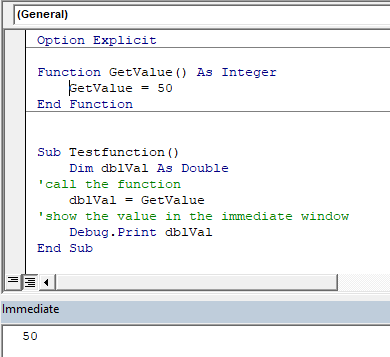The value of 50 would always be returned.

You can also call the GetRange function from a Sub Procedure.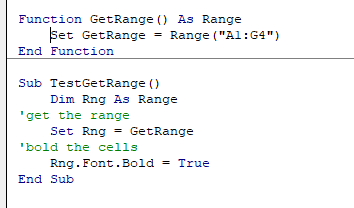In the above example, the GetRange Function is called by the Sub Procedure to bold the cells in the range object.

## Creating Functions

### Single Argument

You can also assign a parameter or parameters to your function.  These parameters can be referred to as Arguments.

``````Function ConvertKilosToPounds (dblKilo as Double) as Double
ConvertKiloToPounds = dblKilo*2.2
End Function``````

We can then call the above function from a Sub Procedure in order to work out how many pounds a specific amount of kilos are.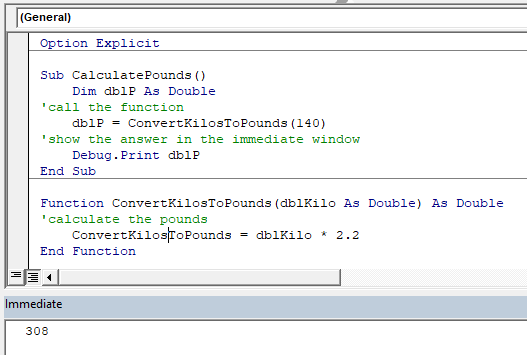A function can be a called from multiple procedures within your VBA code if required.  This is very useful in that it stops you from having to write the same code over and over again.  It also enables you to divide long procedures into small manageable functions.In the above example, we have 2 procedures – each of them are using the Function to calculate the pound value of the kilos passed to them in the dblKilo Argument of the function.

### Multiple Arguments

You can create a Function with multiple arguments and pass the values to the Function by way of a Sub Procedure.

``````Function CalculateDayDiff(Date1 as Date, Date2 as Date) as Double
CalculateDayDiff = Date2-Date1
End Function``````

We can then call the function to calculate the amount of days between 2 dates.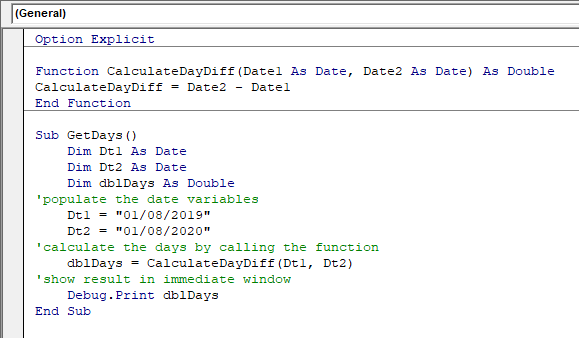### Optional Arguments

You can also pass Optional arguments to a Function.  In other words, sometimes you may need the argument, and sometimes you may not – depending on what code you are using the Function with .

``````Function CalculateDayDiff(Date1 as Date, Optional Date2 as Date) as Double
'check for second date and if not there, make Date2 equal to today's date.
If Date2=0 then Date2 = Date
'calculate difference
CalculateDayDiff = Date2-Date1
End Function``````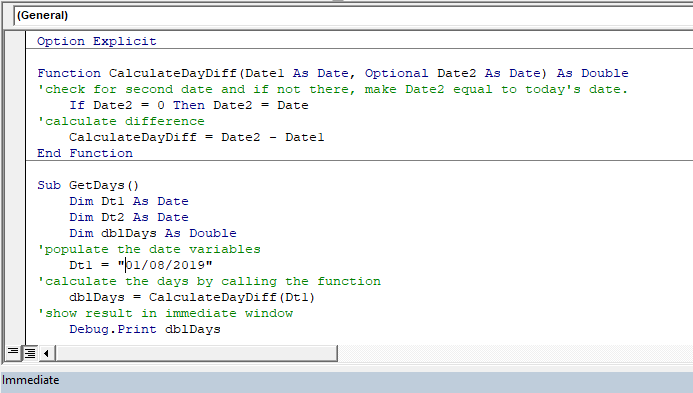## VBA Coding Made Easy

Stop searching for VBA code online. Learn more about AutoMacro - A VBA Code Builder that allows beginners to code procedures from scratch with minimal coding knowledge and with many time-saving features for all users!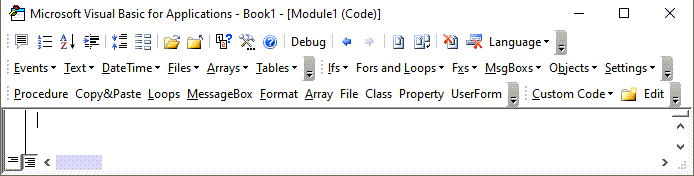### Default Argument Value

You can also set the default value of the Optional arguments when you are creating the function so that if the user omits the argument, the value that you have put as default will be used instead.

``````Function CalculateDayDiff(Date1 as Date, Optional Date2 as Date="06/02/2020") as Double
'calculate difference
CalculateDayDiff = Date2-Date1
End Function``````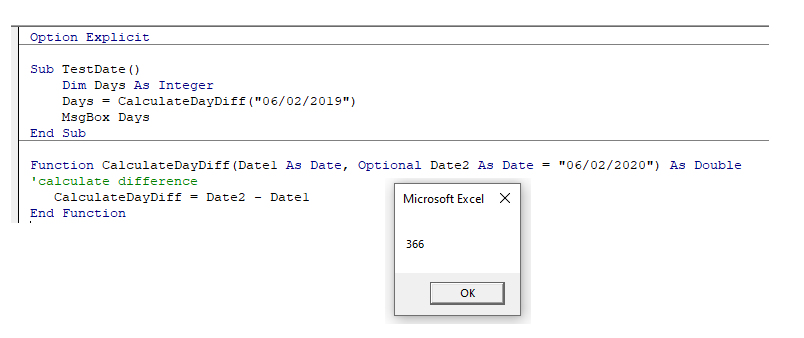### ByVal and ByRef

When you pass values to a function, you can use the ByVal or ByRef keywords.  If you omit either of these, the ByRef is used as the default.

ByVal means that you are passing a copy of the variable to the function, whereas ByRef means you are referring to the original value of the variable.  When you pass a  copy of the variable (ByVal), the original value of the variable is NOT changed, but when you reference the variable, the original value of the variable is changed by the function.

``````Function GetValue(ByRef intA As Integer) As Integer
intA = intA * 4
GetValue = intA
End Function``````

In the function above, the ByRef could be omitted and the function would work the same way.

``````Function GetValue(intA As Integer) As Integer
intA = intA * 4
GetValue = intA
End Function``````

To call this function, we can run a sub-procedure.

``````Sub TestValues()
Dim intVal As Integer
'populate the variable with the value 10
intVal = 10
'run the GetValue function, and show the value in the immediate window
Debug.Print GetValue(intVal)
'show the value of the intVal variable in the immediate window
Debug.Print  intVal
End Sub``````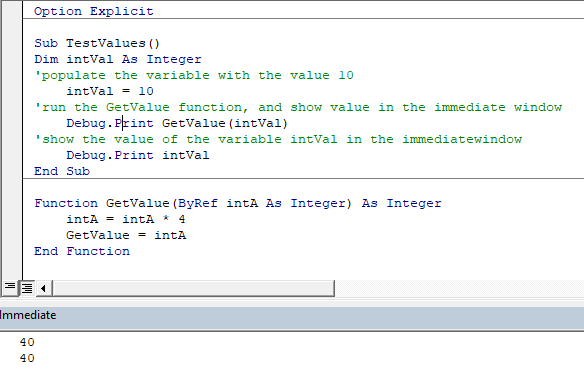Note that the debug windows show the value 40 both times.  When you pass the variable IntVal to the function – the value of 10 is passed to the function, and multiplied by 4.  Using the ByRef keyword (or omitting it altogether), will AMEND the value of the IntVal variable.   This is shown when you show first the result of the function in the immediate window (40), and then the value of the IntVal variable in the debug window (also 40).

If we do NOT want to change the value of the original variable, we have to use ByVal in the function.

``````Function GetValue(ByVal intA As Integer) As Integer
intA = intA * 4
GetValue = intA
End Function``````

Now if we call the function from a sub-procedure, the value of the variable IntVal will remain at 10.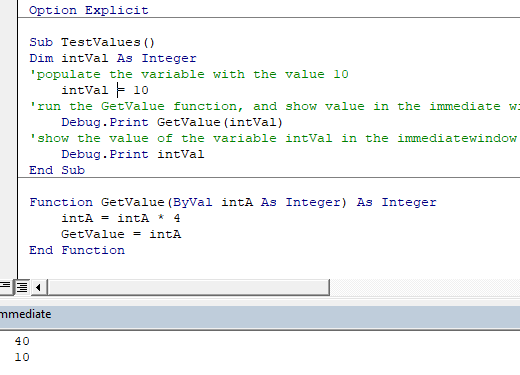## Exit Function

If you create a function that tests for a certain condition, and once the condition is found to be true, you want return the value from the function, you may need to add an Exit Function statement in your Function in order to exit the function before you have run through all the code in that function.

``````Function FindNumber(strSearch As String) As Integer
Dim i As Integer
'loop through each letter in the string
For i = 1 To Len(strSearch)
'if the letter is numeric, return the value to the function
If IsNumeric(Mid(strSearch, i, 1)) Then
FindNumber= Mid(strSearch, i, 1)
'then exit the function
Exit Function
End If
Next
FindNumber= 0
End Function``````

The function above will loop through the string that is provided until it finds a number, and then return that number from the string.  It will only find the first number in the string as it will then Exit the function.

The function above can be called by a Sub routine such as the one below.

``````Sub CheckForNumber()
Dim NumIs as Integer
'pass a text string to the find number function
NumIs = FindNumber("Upper Floor, 8 Oak Lane, Texas")
'show the result in the immediate window
Debug.Print NumIs
End Sub``````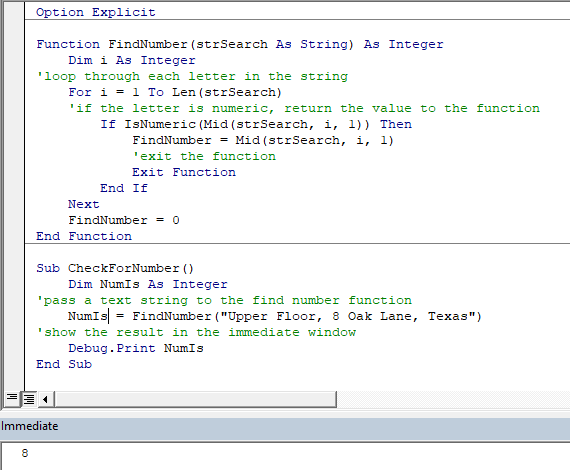VBA Programming | Code Generator does work for you!

## Using a Function from within an Excel Sheet

In addition to calling a function from your VBA code using a sub procedure, you can also call the function from within your Excel sheet.  The functions that you have created should by default appear in your function list in the User Defined section of the function list.

Click on the fx to show the Insert Function dialog box.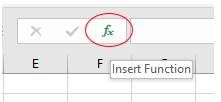Select User Defined from the Category List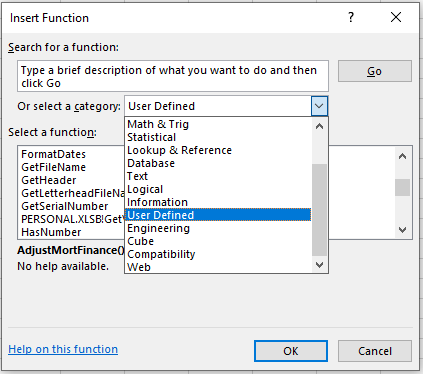Select the function you require from the available User Defined Functions (UDF’s).Alternatively, when you start writing your function in Excel, the function should appear in the drop down list of functions.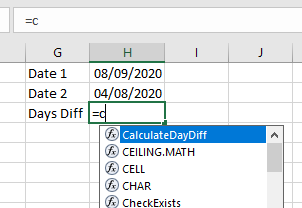If you do not want the function to be available inside an Excel sheet, you need to put the Private word in front of the word Function when you create the function in your VBA code.

``````Private Function CalculateDayDiff(Date1 as Date, Date2 as Date) as Double
CalculateDayDiff = Date2-Date1
End Function``````

It will now not appear in the drop down list showing the Excel functions available.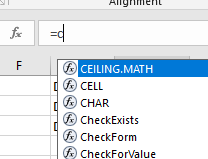Interestingly enough, however, you can still use the function – it just will not appear in the list when looking for it!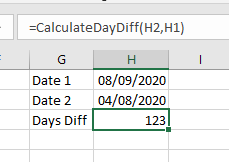If you have declared the second argument as Optional, you can omit it within the Excel sheet as well as within the VBA code.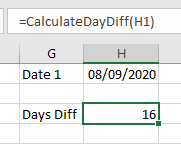You can also use the a function that you have created without arguments in your Excel sheet.